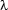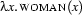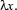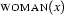## 3.4 The Lambda Calculus

In this section we will dicuss a mechanism that gives us a grip on the gaps in templates like the ones making up the complex `+`-terms that our first approach produced for sentences. We will explicitely mark such gaps and give them names. This will allow us to state precisely how to build a complete first order formula out of a sequence of parts. So we will be able to give a nice and systematic account of the post-processing that used to be done by `insertArgs/3` in our naive approach.

The naive approach to semantic construction discussed in the previous section became infeasible and very inelegant. But where exactly did we go wrong? As we realized when our sentences became more complex, the problem is that up to now we completely lack a uniform way of combining the collected semantic material into well-formed first order formulae.

In this section we introduce-calculus, a solution for this problem. For present purposes we shall view it as a notational extension of first order logic that allows us to bind variables using a new variable binding operator. Here is a simple-expression:The prefixbinds the occurrence ofin. It so gives us a handle on this variable, which we can use to state how and when other symbols should be inserted for it.

Aljoscha Burchardt, Stephan Walter, Alexander Koller, Michael Kohlhase, Patrick Blackburn and Johan Bos
Version 1.2.5 (20030212)